﻿ Northlake Preschool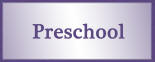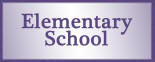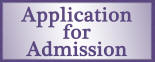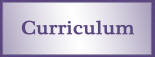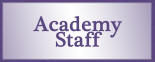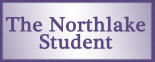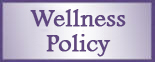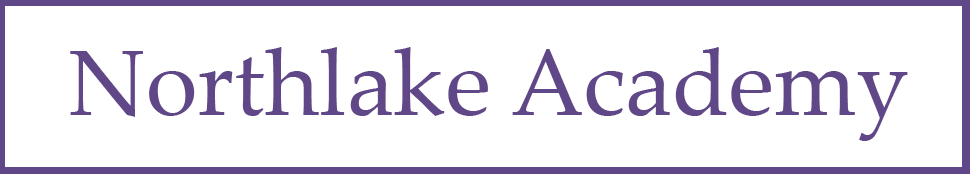Number and Numeration

 Program Goal Content Thread Grade-Level Goal Understand the Meanings, Uses, and Representations of Numbers Rote counting Count on by 1s, 2s, 5s, 10s, 25s, and 100s past 1,000 and back by 1s, 10s, and 100s from any number less than 1,000 with and without number grids, number lines, and calculators. Place value and notation Read, write, and model with manipulatives whole numbers up to 10,000; identify places in such numbers and the values of the digits in those places; read and write money amounts in dollars-and-cents notation. Meanings and uses of fractions Use manipulatives and drawings to model fractions as equal parts of a region or a collection; describe the models and name the fractions. Number theory Recognize numbers as odd or even. Understand Equivalent Names for Numbers Equivalent names for whole numbers Use tally marks, arrays, and numerical expressions involving addition and subtraction to give equivalent names for whole numbers. Equivalent names for fractions, decimals, and percents Use manipulatives and drawings to model equivalent names for 1/2. Understand Common Numerical Relations Comparing and ordering numbers Compare and order whole numbers up to 10,000; use area models to compare fractions.

Operations and Computation

 Program Goal Content Thread Grade-Level Goal Compute Accurately Addition and subtraction facts Demonstrate automaticity with all addition facts through 10 + 10 and fluency with the related subtraction facts. Addition and subtraction procedures Use manipulatives, number grids, tally marks, mental arithmetic, paper & pencil, and calculators to solve problems involving the addition and subtraction of multidigit whole numbers; describe the strategies used; calculate and compare values of coin and bill combinations. Make Reasonable Estimates Computational estimation Make reasonable estimates for whole number addition and subtraction problems; explain how the estimates were obtained. Understand Meanings of Operations Models for the operations Identify and describe change, comparison, and parts-and-total situations; use repeated addition, arrays, and skip counting to model multiplication; use equal sharing and equal grouping to model division.

Data and Chance

 Program Goal Content Thread Grade-Level Goal Select and Create Appropriate Graphical Representations of Collected or Given Data Data collection and representation Collect and organize data or use given data to create tally charts, tables, graphs, and line plots. Analyze and Interpret Data Data analysis Use graphs to ask and answer simple questions and draw conclusions; find the maximum, minimum, mode, and median of a data set. Understand and Apply Basic Concepts of Probability Qualitative probability Describe events using certain, likely, unlikely, impossible, and other basic probability terms; explain the choice of language.

Measurement and Reference Frames

 Program Goal Content Thread Grade-Level Goal Understand the Systems and Processes of Measurement; Use Appropriate Techniques, Tools, Units, and Formulas in Making Measurements Length, weight, and angles Estimate length with and without tools; measure length to the nearest inch and centimeter; use standard and nonstandard tools to measure and estimate weight. Area, perimeter, volume, and capacity Partition rectangles into unit squares and count unit squares to find areas. Units and systems of measurement Describe relationships between days in a week and hours in a day. Money Make exchanges between coins and bills. Use and Understand Reference Frames Temperature Read temperature on both the Fahrenheit and Celsius scales. Time Tell and show time to the nearest five minutes on an analog clock; tell and write time in digital notation.

Geometry

 Program Goal Content Thread Grade-Level Goal Investigate Characteristics and Properties of Two- and Three-Dimensional Geometric Shapes Lines and angles Draw line segments and identify parallel line segments. Plane and solid figures Identify, describe, and model plane and solid figures including circles, triangles, squares, rectangles, hexagons, trapezoids, rhombuses, spheres, cylinders, rectangular prisms, pyramids, cones, and cubes. Apply Transformations and Symmetry in Geometric Situations Transformations and symmetry Create and complete two-dimensional symmetric shapes or designs.

Patterns, Functions, and Algebra

 Program Goal Content Thread Grade-Level Goal Understand Patterns and Functions Patterns and functions Extend, describe, and create numeric, visual, and concrete patterns; describe rules for patterns and use them to solve problems; use words and symbols to describe and write rules for functions involving addition and subtraction and use those rules to solve problems. Use Algebraic Notation to Represent and Analyze Situations and Structures Algebraic notation and solving number sentences Read, write, and explain expressions and number sentences using the symbols +,-, =, >, and <; solve number sentences involving addition and subtraction; write expressions and number sentences to model number stories. Properties of the arithmetic operations Describe the Commutative and Associative Properties of Addition and the Additive Identity and apply them to mental arithmetic problems.